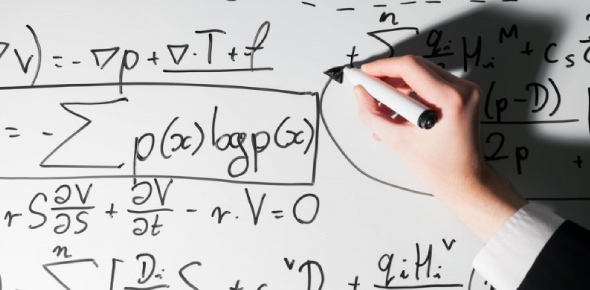# Only Geniuses Can Crack This Maths Quiz

20 Questions | Attempts: 771SettingsThis is a tough maths quiz having algebric and numerical questions! If you pass this test, you are a math master because "Only Geniuses Can Crack This Maths Quiz" Share this quiz on your social media and with your friends to test their mathematical IQ. ALL THE BEST

• 1.
104 equals to 1 followed by how many zeros?
• A.

1

• B.

2

• C.

3

• D.

4

• E.

5

• 2.
25 = [Blank]
• 3.
Fraction is basically same as division.   So this fraction, 48/8 = [Blank]
• 4.
Simplify 18/28 = [Blank]
• 5.
2n = 20, then n = [Blank] (2n means 2 times n)
• 6.
If 3x = 666, then x = [Blank]
• 7.
Simplify 36/63 = [Blank]
• 8.
58 + (22 - 4) x (36 - 34) = [Blank]
• 9.
40 x 63 = [Blank]
• 10.
The probability of rolling an even number on a regular die is [Blank]
• 11.
The probability of rolling 5 on a regular die is [Blank]
• 12.
The probability of rolling 5 OR an even number on a regular die is [Blank]
• 13.
The probability of rolling a 7 with an octahedron die is [Blank]
• 14.
If two angles of an isoceles triangle are 32 degrees each, the third angle is [Blank] degrees.
• 15.
Simplify 11/15 = [Blank]
• 16.
57 + (12 + 41) x (4 - 4) = [Blank]
• 17.
6 + 8 x 10 / 2 - 2 = p   , then p = [Blank] Remember :  the symbol  " / " means divide.  Fraction = Division
• 18.
15 x 2 - 33 = [Blank]
• 19.
Sum of all the angles in an equilateral triangle = [Blank] degrees.
• 20.
A rectangle has [Blank] right angles.

## Related Topics

×

Wait!
Here's an interesting quiz for you.